Ministério da Ciência, Tecnologia, Inovações e Comunicações CBPFIndex CENTRO BRASILEIRO DE PESQUISAS FÍSICAS11/12/2023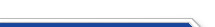Usuário: Senha: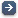Crie sua conta aqui · Busca rápida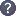Procurar por: Publicações Projetos EventosIr para a busca avançada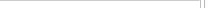Notas de Física
 Inequivalent ${\mathbb Z}_2^n$-graded brackets, $n$-bit parastatistics and statistical transmutations of supersymmetric quantum mechanics Data do cadastro: 19/09/2023 Publicação/Divulgação: 19/09/2023 Resp. pelo cadastro: Status atual: Publicado Ano da publicação: 2023 Descrição: Given an associative ring of ${\mathbb Z}_2^n$-graded operators, the number of inequivalent brackets of Lie-type which are compatible with the grading and satisfy graded Jacobi identities is $b_n= n+\lfloor n/2\rfloor+1$. This follows from the Rittenberg-Wyler and Scheunert analysis of color" Lie (super)algebras which is revisited here in terms of Boolean logic gates. \\The inequivalent brackets, recovered from ${\mathbb Z}_2^n\times {\mathbb Z}_2^n\rightarrow {\mathbb Z}_2$ mappings, are defined by consistent sets of commutators\slash anticommutators describing particles accommodated into an $n$-bit parastatistics (ordinary bosons/fermions correspond to $1$ bit). Depending on the given graded Lie (super)algebra, its graded sectors can fall into different classes of equivalence expressing different types of particles (bosons, parabosons, fermions, parafermions). As a consequence, the assignment of certain marked" operators to a given graded sector is a further mechanism to induce inequivalent graded Lie (super)algebras (the basic examples of quaternions, split-quaternions and biquaternions illustrate these features). \\ As a first application we construct ${\mathbb Z}_2^2$ and ${\mathbb Z}_2^3$-graded quantum Hamiltonians which respectively admit $b_2=4$ and $b_3=5$ inequivalent multiparticle quantizations (the inequivalent parastatistics are discriminated by measuring the eigenvalues of certain observables in some given states). The extension to ${\mathbb Z}_2^n$-graded quantum Hamiltonians for $n>3$ is immediate.\\ As a main physical application we prove that the ${\cal N}$-extended, one-dimensional supersymmetric and superconformal quantum mechanics, for ${\cal N}=1,2,4,8$, are respectively described by $s_{\cal N}=2,6,10,14$ alternative formulations based on the inequivalent graded Lie (super)algebras. The $s_{\cal N}$ numbers correspond to all possible statistical transmutations" of a given set of supercharges which, for ${\cal N}=1,2,4,8$, are accommodated into a ${\mathbb Z}_2^n$-grading with $n=1,2,3,4$ (the identification is ${\cal N}= 2^{n-1}$).\\ In the simplest ${\cal N}=2$ setting (the $2$-particle sector of the de Alfaro-Fubini-Furlan deformed oscillator with $sl(2|1)$ spectrum-generating superalgebra), the ${\mathbb Z}_2^2$-graded parastatistics imply a degeneration of the energy levels which cannot be reproduced by ordinary bosons/fermions statistics. Número: CBPF-NF-002/23 Autores: M.M. Balbino; I.P. de Freitas; R.G. Rana; F. Toppan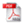Download do PDF (1.846 KB)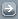Todos EventosTodos PublicaçõesTodasArtigos de Div. CientíficaArtigos em Revistas Nac.Artigos em Revistas Int.Artigos em Eventos Nac.Artigos em Eventos Int.Livros InteirosCapítulos de LivroPropriedades IntelectuaisÁudioPatentesProgramas de computadorModelos de utilidadeMarcasOutras prop. intel.TecnologiasKnow-howServiços tecnológicosTecnologias inovadorasTeses de DoutoradoDissertações de MestradoProjetos de GraduaçãoCiência e SociedadeDocumentos HistóricosMonografiasNotas de FísicaNotas TécnicasOutras Produções
 · Principal    |    CBPF    |    · Copyright 2004 - CBPF. Rio de Janeiro/BrasilCentro Brasileiro de Pesquisas Físicasv 1.1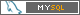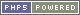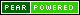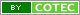Pï¿½g. gerada em 0.01 s Total de consultas SQL: 25